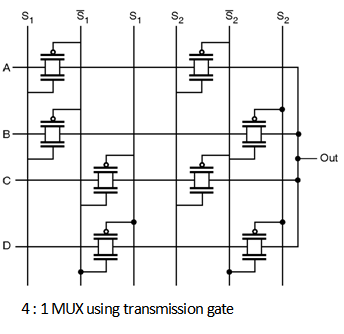Home > Digital CMOS Design > Pass Transistor Logic > 4 1 multiplexer using CMOS logic

4-1-multiplexer_using_CMOS_logic | Pass-Transistor-Logic

4:1 multiplexer using CMOS logic The path selector logic Boolean expression can be given as :

Out = AS + B––S

When the select line signal S is high A is passed to the output and when S is low B is passed to the output. The same logic is used for 4 : 1 MUX in which number of inputs are four (A, B, C, D) and the number of select lines are two (S1, S2). The Boolean expression for 4 : 1 MUX can be given as :

Out = A (S1  S2) + B (S1  ––S2) + C (––S1  S2) + D (––S1  ––S2)

The above Boolean expression can be used to implement 4 : 1 multiplexer or 1 : 4 demultiplexer. The above logic can be generalised as :

2m = n

Where n is the number of inputs in case of MUX (outputs in case of DEMUX) and m is the number of control lines.

4 : 1 MUX using CMOS logic

The implementation of 4 : 1 MUX using CMOS logic is shown in Figure below.

4 : 1 MUX using transmission gates

The implementation of 4 : 1 MUX using transmission gates is shown in

Figure below.4 : 1 MUX using pass transistor logic

The implementation of 4 : 1 MUX using pass transistor logic is as shown in

Figure below.